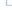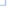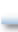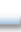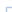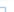# ps_hyphenate

(PECL ps:1.1.1-1.3.5)

ps_hyphenateHyphenates a word

### Description

array ps_hyphenate ( resource \$psdoc , string \$text )

Hyphenates the passed word. ps_hyphenate() evaluates the value hyphenminchars (set by ps_set_value()) and the parameter hyphendict (set by ps_set_parameter()). hyphendict must be set before calling this function.

This function requires the locale category LC_CTYPE to be set properly. This is done when the extension is initialized by using the environment variables. On Unix systems read the man page of locale for more information.

### Parameters

psdoc

Resource identifier of the postscript file as returned by ps_new().

text

text should not contain any non alpha characters. Possible positions for breaks are returned in an array of interger numbers. Each number is the position of the char in text after which a hyphenation can take place.

### Return Values

An array of integers indicating the position of possible breaks in the text or FALSE in case of an error.

### Examples

Example #1 Hyphennate a text

``` <?php\$word = "Koordinatensystem";\$psdoc = ps_new();ps_set_parameter(\$psdoc, "hyphendict", "hyph_de.dic");\$hyphens = ps_hyphenate(\$psdoc, \$word);for(\$i=0; \$i<strlen(\$word); \$i++) {  echo \$word[\$i];  if(in_array(\$i, \$hyphens))    echo "-";}ps_delete(\$psdoc);?> ```

The above example will output:

```Ko-ordi-na-ten-sys-tem

```

### See Also